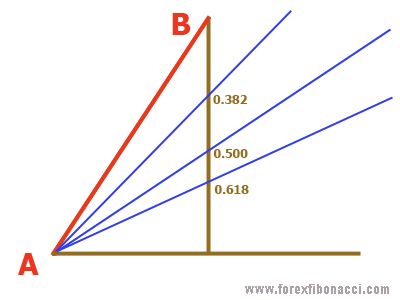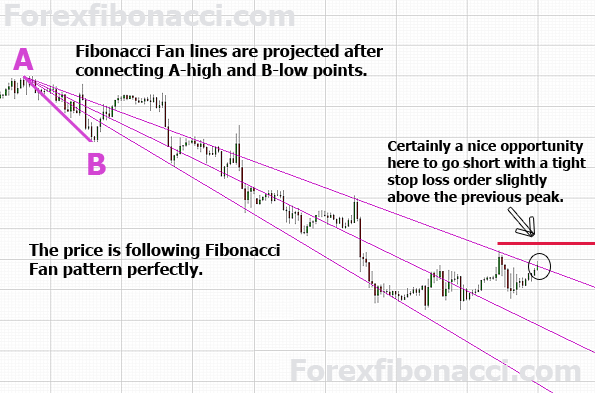# Fibonacci method in Forex

### Forex trading with Fibonacci method 📈 Mini-lesson on how to use FibonacciHOMEFIBONACCI FANCALCULATE FIBONACCI LEVELSFIBONACCI CALCULATOR FIBONACCI FAQ

## Fibonacci Fan

Fibonacci Fan is another Fibonacci retracement tool that takes both price and time into consideration. Comparing to horizontal Fibonacci lines it offers an additional feature — price movement projection far further in time.

The price projection is based on fan-like trend lines that represent already familiar to us Fibonacci numbers: 0.382, 0.500 and 0.618.

That how it looks on the sketch:To place Fibonacci Fan on the chart, choose Fibonacci Fan tool from menu on your trading platform and then look for the highest high and lowest low points (in other words two opposite peaks) at any given time to plot the tool on. Simply click on one peak and drug all the way to the other peak, the trading platform will build a fan for you.

Let's now take a look at the real chart:Fibonacci Fan represents price future support and resistance levels. Traders can expect for the price either to stay in the fan and move in between lines, or break out of it.

When a price holds at any Fan line it indicates presence of support/resistance there. Once the price breaks through the line, it won't usually stop till the next Fan line is met.
If the price quickly passes a Fan line (which means there was no support/resistance there), it will freely move to the next Fan line.

Even trading outside the Fibonacci Fan, price can find strong support/resistance when approaching Fibonacci Fan line.

So, Fibonacci Fan gives traders a simple but effective way to predict future price movements.
However, it is recommended that Fibonacci Fan tool be traded along with other indicators or tools to avoid fake signals.

Jeff Boyd

## RESOURCES

🔎 Forex brokers

📈 Currency Forecasts

🔑 Free Forex strategiesHOMEFIBONACCI FANCALCULATE FIBONACCI LEVELSFIBONACCI CALCULATOR